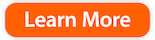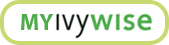Here’s What Students Need to Know About the SAT Math Section

By IvyWise Master Tutor

Understanding test content, formatting, and changes are important when preparing for the SAT – especially the Math section. There have been a number of changes to the SAT math section since the launch of the new exam in 2016, and IvyWise is here to help students better understand how to master this portion of the SAT.

Two Math Sections

The new SAT math section sports a new look: one no-calculator section, one with calculator section. The no-calculator section includes 20 questions timed to 25 minutes; the with-calculator section includes 38 questions in 55 minutes.

This is perhaps the single most significant change from the previous iteration of the SAT Math, and it’s helpful to understand the decision to split the math section into two.

Emphasis on Fundamental Concepts

The first and most important reason for doing so is that it presents the student with a new and unique challenge: the no-calculator section presents questions that would clearly be easier with a calculator. Consider, for instance, the following no-calculator question taken from a released SAT test:A question that could be solved in a flash using a calculator now requires working knowledge of exponent rules.

Emphasis on Efficiency

Conversely, the calculator section does not require such arithmetic work. The College Board readily admits this cruel bit of irony in The Official SAT Study Guide:

“In the calculator portion, many questions do not require a calculator and many questions can be completed faster by hand without using a calculator.”

Students and parents should be aware that the SAT Math sections test not only the ability to execute basic algebraic and arithmetic operations, but also the ability to do them extremely quickly and efficiently.

A strong background in fundamental arithmetic and algebraic skills, such as multiplying or dividing exponents, root numbers, or resolving simultaneous equations, is extremely importnat when preparing for the SAT Math section. Doing so at the pace that the SAT requires will also require practice.

Increased Emphasis on Algebra

But don’t worry, the new SAT Math remains, for the most part, a creature of habit, and retains a lot of the same dynamics as in the previous iteration of the SAT.

Consider, for example, the fact that the SAT, in its past three iterations, has consistently demonstrated a clear emphasis towards algebra topics. That fact has not changed in the new SAT Math:

 Math Section ACT SAT Number of questions 60 58 (total) Time per question 1 minute 1 minute 23 seconds Algebra Content (based on official released tests) 45% 60%

Students that take both tests will notice this important fact – that the SAT puts more of an emphasis on Algebra than the ACT. Now this doesn’t mean that students should decide which test to take solely based on this fact – the ACT has plenty of difficult question types as well – but this is certainly something to keep in mind.

Another important consequence of the SAT’s Algebra emphasis is what it calls “Passport to Advanced Math,” which refers to a host of algebraic concepts that are new to the SAT. However, new does not necessarily mean difficult, just different! ‘Advanced Math’ topics include polynomial division, polynomial factoring, quadratic formula – in other words, topics that students do cover in standard Algebra class.

Students preparing for the SAT math section should work specifically to better their familiarity with fundamental Algebraic concepts.

Again, it’s important to understand the content and formatting of each section when preparing for the SAT. Hopefully, before you start your test prep, you have taken a diagnostic of both the SAT and ACT determined which test is the best fit for your needs and abilities and in which sections you need to improce. Not sure about the differences between each exam? Check out our SAT vs. ACT guide here.